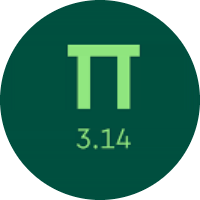# Learning the One Variable Inequalities

In this quiz, You will get to know about the One Variable Inequalities. You will find practice problems of finding the various inequalities. You will learn about the variable Inequalities from the different questions.

Start Quiz

Which of the following is a solution to the inequality below?

y ≤ 3

y = 6

y = 7

y = 12

y = 1

Solve for v.

v/2 ≤ 5

v ≤ 10

v ≤ 15

v ≤ 5

Is t = –2 a solution to the inequality below?

t < –10

yes

no

Solve for k.

k + 4 > 7

k < 3

k > 3

k > 7

Solve for t.

10t + 4 ≤ -6

t ≤ -2

t ≤ -6

t ≤ -1

Solve for n.

2n - 4 ≥ -10

n ≥ -3

n ≥ -1

n ≥ 3

Solve for v.

5 ≤ - 4v - 3

v ≤ 2

v ≤ -10

v ≤ -5

v ≤ -2

Solve for a.

(-a/9) - 3 ≤ -4

a ≥ 5

a ≥ 9

a ≥ 6

Solve for r.

-7r ≤ -6r - 10

r ≥ 10

r ≥ 5

r ≥ 5

r ≥ 7

Solve for j.

-6j ≤ -5 - 7j

j ≤ 5

j ≤ 2

j ≤ -5

Quiz/Test Summary
Title: Learning the One Variable Inequalities
Questions: 10
Contributed by: